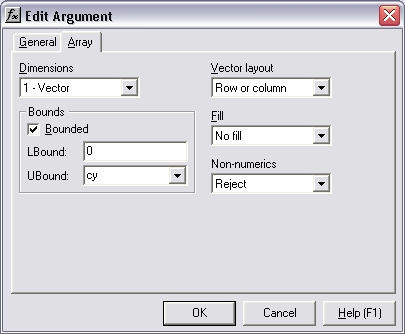HOW TO: How can I make sure that the size of a matrix input matches two vector inputs?

Reference: Q0039

• XLL+ for Visual Studio 2005 - 5.0
• XLL+ for Visual Studio .NET - 4.2, 4.3.1, 5.0
• XLL+ for Visual Studio 6 - 3, 4.1, 4.2, 4.3.1, 5.0

HOW TO: How can I make sure that the size of a matrix input matches two vector inputs?

Question

I have an add-in function with 3 inputs, x, y and z. x and y are vectors, while z is a matrix. The size of z is constrained such that:

1. Height of z = Size of x
2. Width of z = Size of y

You can modify your arguments to be bounded input arrays. The XLL+ Function Wizard has a tab which lets you set the bounds of an array, so that they are tied to a named variable (or to a constant). The code which is generated will automatically check that all matrix and vector inputs satisfy the constraints you apply.

By using the same named variable for each pair of inputs, you can ensure that each dimension of each input is the same size as its pair.

If the input is not of the expected size, then an error value is returned, e.g.:

#Error: expected 5 columns in z

Example

Problem: constrain a matrix input to match the size of two vector inputs.

Steps

1. Create a new function, ConstrainedMatrix(), using the XLL+ Function Wizard. It takes a three arguments: x, y and z. x and y are defined as vectors of doubles. z is defined as a matrix of doubles.2. Select the Dimensions column of the row containing the definition of x, and click on the ... button. (Or use the short-cut Ctrl+E.) This will display the Edit Argument dialog.

Select the Array tab

In the Bounds box, check the Bounded check-box and set the upper bound (UBound) to be cx, as shown below.3. Press OK to close the dialog.
4. Select the Dimensions column of the row containing the definition of y, and click on the ... button. (Or use the short-cut Ctrl+E.) This will display the Edit Argument dialog.

Select the Array tab

In the Bounds box, check the Bounded check-box and set the upper bound (UBound) to be cy, as shown below.5. Press OK to close the dialog.
6. Select the Dimensions column of the row containing the definition of z, and click on the ... button. (Or use the short-cut Ctrl+E.) This will display the Edit Argument dialog.

Select the Array tab

In the Rows box, check the Bounded check-box and set the upper bound (UBound) to be cx, as shown below. This forces the height of z to match the size of x, as required.

In the Columns box, check the Bounded check-box and set the upper bound (UBound) to be cy, as shown below. This forces the height of z to match the size of y, as required.7. Press OK to close the dialog.
8. Inspect the changes to the Function Wizard. The Dimensions column now contains the new definitions of x, y and z.

Argument Dimensions
x Vector (0 to cx)
y Vector (0 to cy)
z Matrix (0 to cx)(0 to cy)9. Press OK to close the dialog, and save the function.

Code

The code generated is shown below:

// Function:    ConstrainedMatrix
// Purpose:     Matrix input is constrained by two vector inputs

//{{XLP_SRC(ConstrainedMatrix)
// NOTE - the FunctionWizard will add and remove mapping code here.
//    DO NOT EDIT what you see in these blocks of generated code!
IMPLEMENT_XLLFN2(ConstrainedMatrix, "RPPP", "ConstrainedMatrix",
"x,y,z", "User Defined", "Matrix input is constrained by two"
" vector inputs", "No description provided\000No description"
" provided\000No description provided\000", "B0(0,cx)x No des"
"cription provided\0B0(0,cy)y No description provided\0B0(0"
",cx)0(0,cy)z No description provided\0appscope=1\0", 1)

extern "C" __declspec( dllexport )
LPXLOPER ConstrainedMatrix(const COper* x, const COper* y, const
COper* z)
{
XLL_FIX_STATE;
CXlOper xloResult;
BOOL bOk = TRUE;
long cx = -1;
long cy = -1;
std::vector<double> vecx;
bOk = bOk && x->ReadVectorBounded(vecx, "x", xloResult, 0, cx);
std::vector<double> vecy;
bOk = bOk && y->ReadVectorBounded(vecy, "y", xloResult, 0, cy);
MTX_PTRS<double> matz;
bOk = bOk && z->ReadMatrix(matz, "z", xloResult, &cx, &cy, XLA_ARRAY_FLAGS_NUMERIC_STD, 0, 0);
if (!bOk)
return xloResult.Ret();
//}}XLP_SRC

// TODO - Set the value of xloResult
return xloResult.Ret();
}

Explanation

The interesting code is shown in bold above.

1. The bounds variables cx and cy, which we entered as UBounds in the Edit Argument dialog, are declared, and are each initialized to -1. This value represents "not yet known".
2. A reference to cx is passed to COper::ReadVectorBounded when reading x, and is also passed to COper::ReadMatrix (as lpUBound1) when reading z.
3. When ReadVectorBounded receives cx, it contains -1 (meaning "not yet known"), and ReadVectorBounded simply sets its value to the size of x.
4. When ReadMatrix is called, cx contains a non-negative integer, and is therefore treated as a fixed size constraint. If z does not contain cx rows then an error is returned.
5. Similarly, a reference to cy is also passed to ReadVectorBounded (when reading y) and to ReadMatrix, as lpUBound2 (when reading z). An error is returned if the width of z does not match the length of y.

Result

If, for example, x contains 6 cells, y contains 4 cells and z contains 6 rows x 5 columns , then the following error value is returned

#Error: expected 4 columns in z

and the function does not continue.